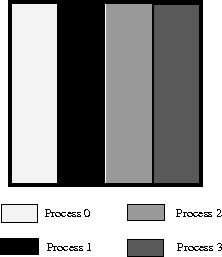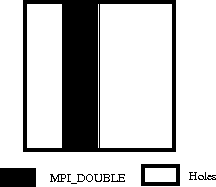## 279. Subarray Filetype ConstructorUp: Examples Next: Profiling Interface Previous: Double Buffering with Split Collective I/OFigure 28: Example array file layoutFigure 29: Example local array filetype for process 1

Assume we are writing out a 100x100 2D array of double precision floating point numbers that is distributed among 4 processes such that each process has a block of 25 columns (e.g., process 0 has columns 0-24, process 1 has columns 25-49, etc.; see Figure 28 ). To create the filetypes for each process one could use the following C program (see Section Subarray Datatype Constructor on page Subarray Datatype Constructor ):

```   double subarray;
MPI_Datatype filetype;
int sizes, subsizes, starts;
int rank;

MPI_Comm_rank(MPI_COMM_WORLD, &rank);
sizes=100; sizes=100;
subsizes=100; subsizes=25;
starts=0; starts=rank*subsizes;

MPI_Type_create_subarray(2, sizes, subsizes, starts, MPI_ORDER_C,
MPI_DOUBLE, &filetype);
```
Or, equivalently in Fortran:

```       double precision subarray(100,25)
integer filetype, rank, ierror
integer sizes(2), subsizes(2), starts(2)

call MPI_COMM_RANK(MPI_COMM_WORLD, rank, ierror)
sizes(1)=100
sizes(2)=100
subsizes(1)=100
subsizes(2)=25
starts(1)=0
starts(2)=rank*subsizes(2)

call MPI_TYPE_CREATE_SUBARRAY(2, sizes, subsizes, starts, &
MPI_ORDER_FORTRAN, MPI_DOUBLE_PRECISION,       &
filetype, ierror)
```
The generated filetype will then describe the portion of the file contained within the process's subarray with holes for the space taken by the other processes. Figure 29 shows the filetype created for process 1.Up: Examples Next: Profiling Interface Previous: Double Buffering with Split Collective I/O